# Student test-test

A significance test for the mean value of a normal distribution.

## The single-sample Student test.

Let the independent random variablesbe subject to the normal law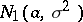, the parametersand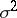of which are unknown, and let a simple hypothesis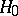: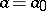be tested against the composite alternative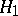: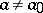. In solving this problem, a Student test is used, based on the statistic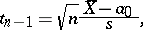where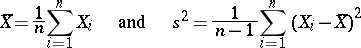are estimators of the parametersand, calculated with respect to the sample. Whenis correct, the statistic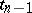is subject to the Student distribution withdegrees of freedom, i.e.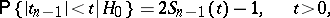whereis the Student distribution function withdegrees of freedom. According to the single-sample Student test with significance level,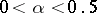, the hypothesismust be accepted if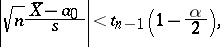where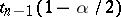is the quantile of levelof the Student distribution with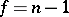degrees of freedom, i.e.is the solution of the equation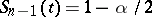. On the other hand, if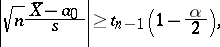then, according to the Student test of level, the tested hypothesis:has to be rejected, and the alternative hypothesis:has to be accepted.

## The two-sample Student test.

Letand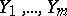be mutually independent normally-distributed random variables with the same unknown variance, and let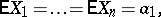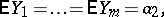where the parameters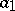and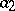are also unknown (it is often said that there are two independent normal samples). Moreover, let the hypothesis: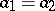be tested against the alternative: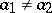. In this instance, both hypotheses are composite. Using the observationsandit is possible to calculate the estimators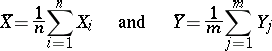for the unknown mathematical expectationsand, as well as the estimatorsfor the unknown variance. Moreover, let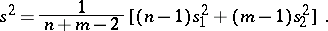Then, whenis correct, the statistic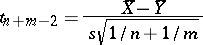is subject to the Student distribution withdegrees of freedom. This fact forms the basis of the two-sample Student test for testingagainst. According to the two-sample Student test of level,, the hypothesisis accepted if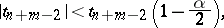where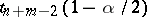is the quantile of levelof the Student distribution with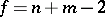degrees of freedom. If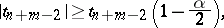then, according to the Student test of level, the hypothesisis rejected in favour of.